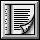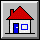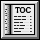5.7 Class CHARACTER`indexing`
`description: "Characters, with comparison operations and an ASCII code"`
`expanded class interface`
`CHARACTER`
`feature -- Access`
```code: INTEGER
-- Associated integer value```
```hash_code: INTEGER
-- Hash code value
-- (From HASHABLE.)
ensure
good_hash_value: Result >= 0```
`feature -- Comparison`
```infix "<" (other: like Current): BOOLEAN
-- Is other greater than current character?
-- (From COMPARABLE.)
require
other_exists: other /= Void
ensure
asymmetric: Result implies not (other < Current)```
```infix "<=" (other: like Current): BOOLEAN
-- Is current character less than or equal to other?
-- (From COMPARABLE.)
require
other_exists: other /= Void
ensure
definition: Result = (Current < other) or is_equal (other);```
```infix ">=" (other: like Current): BOOLEAN
-- Is current object greater than or equal to other?
-- (From COMPARABLE.)
require
other_exists: other /= Void
ensure
definition: Result = (other <= Current)```
```infix ">" (other: like Current): BOOLEAN
-- Is current object greater than other?
-- (From COMPARABLE.)
require
other_exists: other /= Void
ensure
definition: Result = (other < Current)```
```max (other: like Current): like Current
-- The greater of current object and other
-- (From COMPARABLE.)
require
other_exists: other /= Void
ensure
current_if_not_smaller: (Current >= other) implies (Result = Current)
other_if_smaller: (Current < other) implies (Result = other)```
```min (other: like Current): like Current
-- The smaller of current object and other
-- (From COMPARABLE.)
require
other_exists: other /= Void
ensure
current_if_not_greater: (Current <= other) implies (Result = Current)
other_if_greater: (Current > other) implies (Result = other)```
```three_way_comparison (other: like Current): INTEGER
-- If current object equal to other, 0; if smaller,
-- -1; if greater, 1.
-- (From COMPARABLE.)
require
other_exists: other /= Void
ensure
equal_zero: (Result = 0) = is_equal (other);
smaller: (Result = -1) = Current < other;
greater_positive: (Result = 1) = Current > other```
`feature -- Output`
```out: STRING
-- Printable representation of character
-- (From GENERAL.)```
`invariant`
`irreflexive_comparison: not (Current < Current)`
`end`

 Copyright © 1995, Nonprofit International Consortium for Eiffel mailto:nice@atlanta.twr.com Last Updated: 26 October 1997